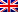# Corrected exercises: time complexity

Contenus

## Corrected exercises about Time complexity

The following corrected exercises are about algorithm analysis, especially correctness, completeness and time complexity calculus.

## Exercise 1

Determine the time complexity of the Check algorithm:Check correctness and completeness, especially what happened during the first iteration.

## Exercise 2

Analyze the complexity of Algorithm. Write another algorithm that does exactly the same thing as Algorithm but with a strictly better asymptotic time complexity.Complexity is O(|A|²). You can improve the algorithm by: first, sort the table in O(n log n) and then check occurrences in O(n), thus complexity becomes O(n log n).

## Exercise 3

What is the (asymptotic) running time of each of the following algorithms, as a function of n? Justify your answers.

a)

for i = 1 to n do

for j = 1 to 2n + 1 do

print (« Hello World)

end for

end for

b)

for i = 1 to 10 do

for j = 1 to n do

print (« Hello World »)

end for

end for

c)

for i = 1 to n do

for j = i to n do

print (« Hello World »)

end for

end for

d)

for i = 1 to n do

for j = 1 to 2 ∗ i + 1 do

print (« Hello World »)

end for

end for

e)

for i = 1 to n ∗ n do

for j = 1 to i do

print (« Hello World »)

end for

end for

f)

for (i = 0 to m) do

t ← 1

while (t < m) do

print (« Hello world »)

t ← t ∗ 2

end while

end for

• a) O(n²)
• b) O(n)
• c) O(n²)
• d) O(n²)
• e) O(n4)
• f) O(m log(m))

## Exercise 4

Let TA(n), TB(n) and TC(n) denote the running time of algorithms A, B and C, respectively.

Instruction A

if (n < 100) then

Instruction B

else

for (j = 1 to n) do

Instruction C

end for

end if

What is the running time of this algorithm, under each of the following sets of assumptions? Justify your answers.

1. TA(n) = O(n), TB(n) = O(n2) and TC(n) = O(log n).
2. TA(n) = O(n2), TB(n) = O(n2) and TC(n) = O(log n).
3. TA(n) = O(n2), TB(n) = O(n3) and TC(n) = O(log n).

Since large values of n determine the asymptotic running time, we can neglect the if part of the if statement. Therefore, in all cases, the running time of this algorithm is O(TA(n) + nTC(n)).

1. a) O(n log n)
2. b) O(n²)
3. c) O(n²)

## Exercise 5

What is the worst-case complexity of the each of the following code fragments?

Two loops in a row:

1. for (i = 0; i < N; i++) {
2. sequence of statements}
3. for (j = 0; j < M; j++) {
4. sequence of statements}

How would the complexity change if the second loop went to N instead of M?

A nested loop followed by a non-nested loop:

1. for (i = 0; i < N; i++) {
2. for (j = 0; j < N; j++) {
3. sequence of statements
4. }}
5. for (k = 0; k < N; k++) {
6. sequence of statements}

A nested loop in which the number of times the inner loop executes depends on the value of the outer loop index:

1. for (i = 0; i < N; i++) {
2. for (j = N; j > i; j–) {
3. sequence of statements
4. }}
1. The first loop is O(N) and the second loop is O(M). Since you don’t know which is bigger, you say this is O(N+M). This can also be written as O(max(N,M)). In the case where the second loop goes to N instead of M the complexity is O(N). You can see this from either expression above. O(N+M) becomes O(2N) and when you drop the constant it is O(N). O(max(N,M)) becomes O(max(N,N)) which is O(N).
2. The first set of nested loops is O(N2) and the second loop is O(N). This is O(max(N2,N)) which is O(N2).
3. This is very similar to our earlier example of a nested loop where the number of iterations of the inner loop depends on the value of the index of the outer loop. The only difference is that in this example the inner-loop index is counting down from N to i+1. It is still the case that the inner loop executes N times, then N-1, then N-2, etc, so the total number of times the innermost « sequence of statements » executes is O(N2).
1. Give an analysis of the running time (Big-Oh notation) for each of the following 4 program fragments. Note that the running time corresponds here to the number of times the operation sum++ is executed. sqrt is the function that returns the square root of a given number.1. If it takes 10ms to run program (b) for n=100, how long will it take to run for n=400 ?
2. If it takes 10ms to run program (a) for n=100, how large a problem can be solved in 40ms ?

A and b in O(sqrt(n)), c and d in O(n^5)

With cross product: sqrt(100)=x and y=10ms; sqrt(400)=2x take 20ms

In 40ms, a can run n=1600.FRFRENRUES